# Cartesian Plane

In Mathematics, the cartesian plane is defined as a two-dimensional coordinate plane, which is formed by the intersection of the x-axis and y-axis. The x-axis and y-axis intersect perpendicular to each other at the point called the origin. In this article, we are going to discuss what is a cartesian plane, and the different parts of the cartesian plane with many solved examples.

## Cartesian Plane Definition

The cartesian plane is a two-dimensional coordinate plane formed by the intersection of two perpendicular lines. The horizontal line is known as X-axis, and the vertical line is known as Y-axis. The coordinate point (x, y) on the Cartesian plane says that the horizontal distance of the point from the origin is x, and the vertical distance is y. If the sign of x is positive, the point is on the right of the origin; else it is on the left. Similarly, if the sign is positive for y, the point is y points above the origin else it is y points below it.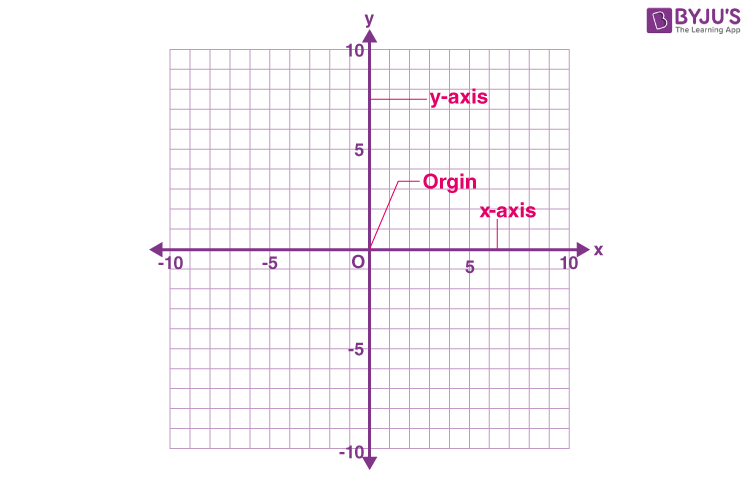Axes

In the cartesian plane, there are two axes. One is the horizontal axis, called the x-axis and the other is a vertical axis, which is called the y-axis.

Origin

In the cartesian plane, the point at which the x-axis and y-axis intersect is called the origin. In the cartesian plane, the origin is represented by an ordered pair or the cartesian coordinate (0, 0).

The X-axis and Y-axis split the plane into four parts, and they are called quadrants. Quadrants are denoted as I, II, III, and IV in an anticlockwise direction. The axes in the plane are called Cartesian axes, and the plane is known as the Cartesian plane.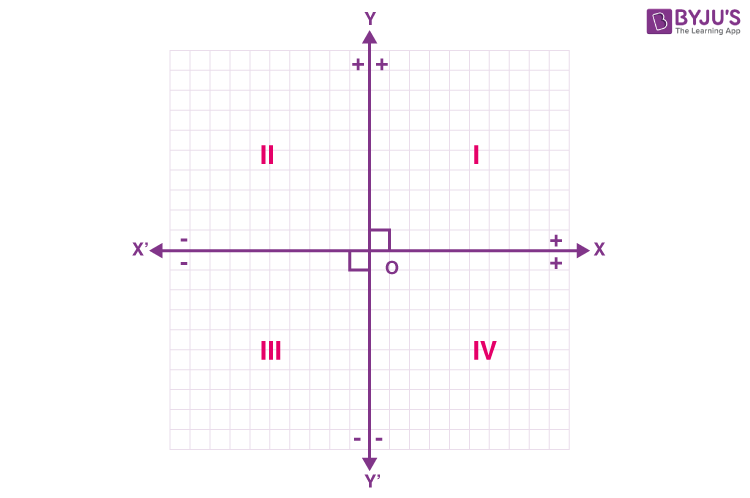Take, the ray

OX = Positive x-axis,

OX’ = Negative x-axis

OY = Positive y-axis

OY’ = Negative y-axis.

Thus the quadrants are characterized by the following signs of abscissa and ordinate:

• Quadrant x > 0 , y > 0 or (+,+)
• Quadrant x < 0 , y > 0 or (-,+)
• Quadrant x < 0 , y < 0 or ( -,-)
• Quadrant x > 0 , y < 0 or (+,-)

The points which lie on the axis, have any one of the coordinates form, such as (x, 0) or (0, y). If the coordinate is of the form (x, 0), then the point is on the x-axis, and if the coordinate is of the form (0, y), then the point is on the y-axis. In this case, the point is not located in any of the quadrants.

Note:

Cartesian Coordinates

The ordered pair in the cartesian plane is called the cartesian coordinates. The cartesian coordinates are of the form  (x, y). The ordered pairs in the two-dimensional plane are referred to as the abscissa and ordinate.

Abscissa: The first number in the ordered pair is called the abscissa. In other words, the value of x in the ordered pair is called the abscissa. It is the distance of any point on the plane from the y-axis.

Ordinate: The second number in the ordered pair is called the ordinate. It takes the value of y. The ordinate is the distance of any point on the plane from the x-axis.

For example, if the cartesian coordinate is (2, 3), 2 represents the abscissa and 3 represents the ordinate.

## One Dimensional Plane

For the Cartesian coordinate system in one dimension, draw a straight line and choose a point O as the origin in the middle of the line. The line segment, which is in the right of the origin O is positive, whereas the line segment in the left of the origin is negative. So give the sign as ‘+’ and ‘-’ to any point as we locate it on the number line. The line, which is chosen for determining the points in one dimension, is called the number line.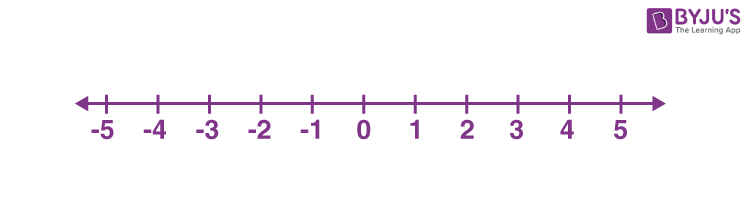Origin:

The centre point from which the distances are marked is called the origin. In two- dimensional plane, the X-axis, and Y-axis crossed the point is called the origin.

## Three Dimensional Plane

The 3D Cartesian plane has one more axis perpendicular to the normal Cartesian plane. For the XY plane, there is an axis Z which is perpendicular to the XY plane. Any point lying on this plane is defined by the set of three points (x, y, z). Here, x represents the position along the X-axis, y defines the position along the Y-axis, and z denotes the position along the Z-axis. The given graph shows the 3D Cartesian plane with three axes X, Y, and Z.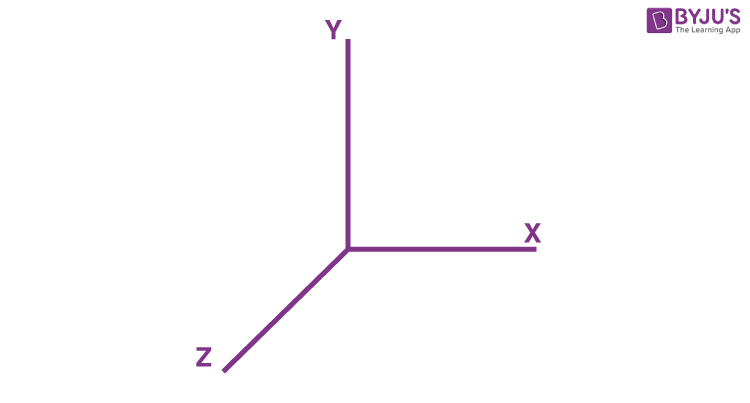### Cartesian Representation of Complex Numbers

A complex number is a blend of real and imaginary numbers. We know that an imaginary number cannot be represented on a number line or Cartesian plane. Hence, they are represented on a complex plane. A complex plane is just like any other two-dimensional Cartesian plane. But here one axis will represent the real part of the number and the other axis will represent the imaginary part of the number. For a complex number a + ib, the point represented on the complex plane will be (a, b).

For example: Take a number 2 + 5i. The given graph will be as given here.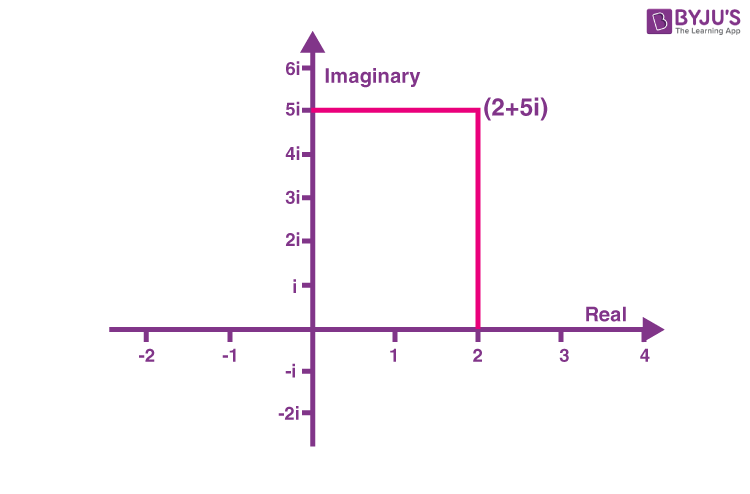### How to Plot the points in the Cartesian plane?

Let us take a cartesian coordinate (2, 3).

To plot the point (2, 3) in the cartesian plane,

• First, identify the abscissa (x-value) and ordinate (y-value) from the given ordered pair. Here, Abscissa = 2 and ordinate= 3.
• Next, plot the value of x (i.e.) “2” on the x-axis. Plot the number, and move 2 unit from the origin to the right, as its value of x is positive.
• Next, plot the value of y, (i.e.) “3” on the y axis. Plot the number, and move 3 units from the origin to the up on the y-axis, as it takes the positive value.

Finally, the point (2, 3) is located on the first quadrant of the cartesian plane.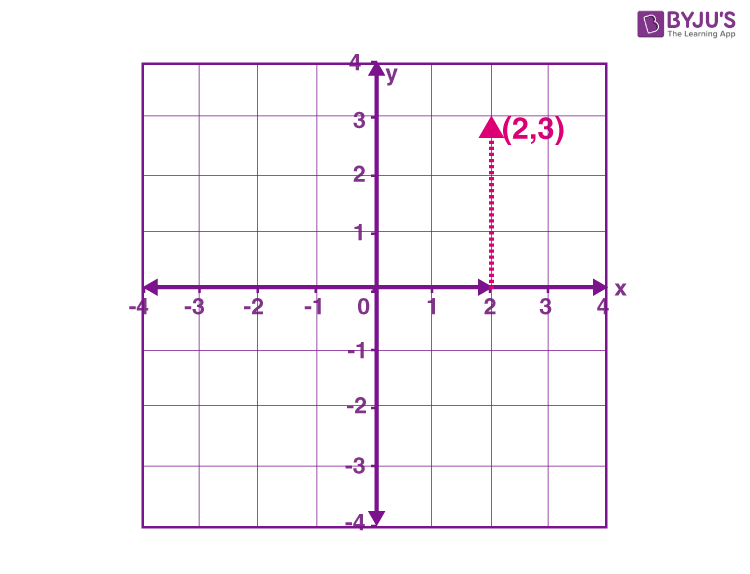Related Links Coordinate System Vertical Line Complex Numbers Real Numbers

### Practice Problems on Cartesian Plane

Graph the following ordered pairs in the cartesian plane:

1. (7, 4)
2. (-5, 2)
3. (4, -5)
4. (-6, -3)

## Frequently Asked Questions on Cartesian Plane

Q1

### What is meant by the cartesian plane?

In Mathematics, a cartesian plane is a two-dimensional coordinate plane, which is formed by the intersection of two lines called x-axis and y-axis.

Q2

### What is meant by abscissa and ordinate?

In cartesian coordinates, the term abscissa represents the x-coordinate value and the term ordinate represents the y-coordinate value.

Q3

### What is meant by origin in the cartesian plane?

The origin is an ordered pair of (0, 0), where the x-axis and y-axis intersect in the cartesian plane.

Q4

### Does the point (-3, -4) lie in the third quadrant?

Yes, the point (-3, -4) lies in the third quadrant. As, both the values of x and y takes the negative value, the point should be located in the third quadrant.

Q5

### Do the x-axis and y-axis intersect perpendicular to each other in the cartesian plane?

Yes, the x-axis and y-axis intersect perpendicular to each other in the cartesian plane, and it makes the four quadrants.

Quiz on Cartesian Plane